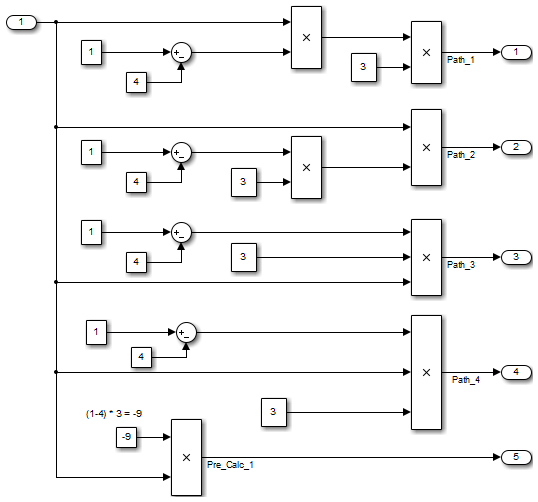## cgsl_0103: Precalculated signals and parameters

ID: Titlecgsl_0103: Precalculated signals and parameters
DescriptionPrecalculate invariant parameters and signals by doing one of the following:
AManually precalculate the values
B

Set these configuration parameters:

• Set Default parameter behavior to `Inlined`

• Select Inline invariant signals

NotesPrecalculating variables can reduce local and global memory usage and improve execution speed. If you set Default parameter behavior to `Inlined` and enable Inline invariant signals, the code generator minimizes the number of run-time calculations by maximizing the number calculations completed before run time. In some cases, this can lead to a reduction in the number of parameters stored. However, the algorithms the code generator uses have limitations. In some cases, the code is more compact if you calculate the values outside of the Simulink® environment. This can improve model efficiency, but can reduce model readability.
RationaleA, BPrecalculate data, outside of the Simulink environment, to reduce memory requirements of a system and improve run-time execution.
Last ChangedR2012b
Examples

In the following model, the four paths are mathematically equivalent. However, due to algorithm limitations, the number of run-time calculations for the paths differs.```Path_1 = InputSignal * -3.0 * 3.0; /* Product: '<Root>/Product4' incorporates: * Inport: '<Root>/In1' */ Path_2 = InputSignal * -9.0; /* Product: '<Root>/Product2' incorporates: * Constant: '<Root>/Constant2' * Inport: '<Root>/In1' */ Path_3 = -9.0 * InputSignal; /* Product: '<Root>/Product5' incorporates: * Constant: '<Root>/Constant2' * Inport: '<Root>/In1' */ Path_4 = -3.0 * InputSignal * 3.0; /* Product: '<Root>/Product6' incorporates: * Constant: '<Root>/Constant3' * Inport: '<Root>/In1' */ Pre_Calc_1 = -9.0 * InputSignal;```

To maximize automatic precalculation, add signals at the end of the set of equations.

Inlining data reduces the ability to tune model parameters. You should define parameters that require calibration to allow calibration. For more information, see Create Tunable Calibration Parameter in the Generated Code (Simulink Coder).